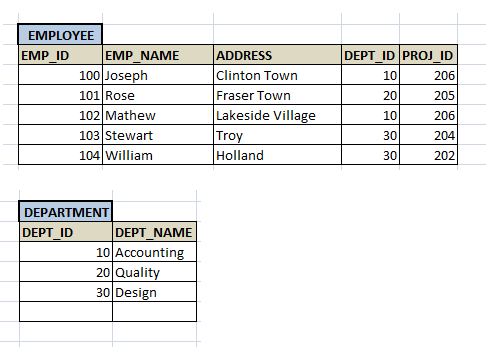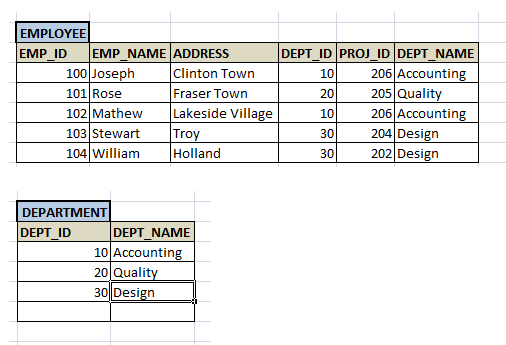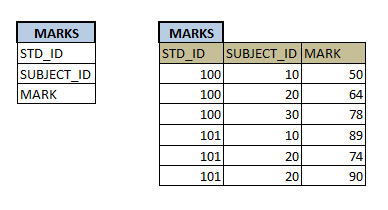# De-normalization in Database

## Introduction

We have seen how to design a database and how to have normalized tables in database. The main purpose of having clear design and normalization of tables is to reduce redundancy and to have consistent data in the database. Hence we will have multiple tables in the database and each will be mapped with one another by referential integrity. If we need any related data we would join the related tables and get the records. This will work fine and quick if the database is small and have relatively few records.

But in real world, the database is very huge and it will have lots of records. When we normalize the table, we might not realize about the record amount. We would be concentrating only on having perfect database with less redundancy. But what happens when data grows in the database? How will a smallest request which has to retrieve the data from multiple tables because of normalization perform? The cost of the query will increase drastically. This is because, it will have to join multiple tables to get the data, but these tables are not smaller. They will huge data, and any smallest query on the table will have to traverse the table till it gets the record (although it depends on file organization method).

For example, in our STUDENT database, we have divided, Address into separate table as Door#, Street, City, State, ZIP. Imagine, if we have to show the address of a student in some report, we always have to have join with this address table. Our query will fetch student’s details from STUDENT table. Once it got the records, it will fetch his address from ADDRESS table. Here the two transaction on fetching and hence disk I/O happens. Hence the cost of performance is more.

Instead, imagine what will happen if we have address in the STUDENT table itself? Second transaction above is not all required. The first fetch itself will give student detail as well as his address. So it saves the second fetch time, making the fetch faster. But what happens to redundancy and 3NF here?

As a thumb rule of database design, we should have normalized data so that there should not be any redundancy. But this normalization increases the cost of performance. Comparing the good design and performance of database, performance takes the priority. Any user accessing the database is more interested in quick and correct result than how a database is designed. Hence if we introduce redundancy in the table and if the performance of the query increases, then we can ignore 3NF.

This process is known as denormalization. In this process, a normalized table will be rejoined to have the redundancy in the table to increase the performance of the query. Denormalization need not be true in all cases. It all depends on the data. Hence this task is performed after the design and after having the data in the tables. In addition, it also depends on the redundant column which we are re-introducing into the table and frequency of this column update. The basic criteria for denormalization would be

• It should reduce the frequency of joins between the tables, and hence making the query faster. If any of the two or more tables are joined often to query the data and this joins costs more, we can combine them into one table. But after combining the table, it should still retain the correct data. There should not be any unwanted/unnecessary duplicate records. In our example above, after denormalization of STUDENT and ADDRESS, it should have all the students with correct address. It should not lead to wrong address of students.
• Most of the cases, when we have joins on tables, full table scan is performed to fetch the data. Hence if the tables are huge, we can think of denormalization.
•  The column should not be updated more frequently. If the columns are updated often, then the cost of update will increase, even though retrieval cost reduces. If it is less frequently updated, then database can bear the cost of update. Otherwise, database will always be hanging. In our case above, address is less frequently updated field (the frequency of a student changing his house is comparatively less). Also the column should very small to get rejoined with the table. Huge columns are again overhead to the table and cost of performance.
• The developer should have very good knowledge of data, when he denormalizes it. He should know very clearly about above factors, frequency of joins / access, updates, column and table size etc.

Denormalization is not only recombining the columns to have redundant data. Denormalization can be any technique with which performance of the normalized table can be increased.

# Methods of De-normalization

There are few of denormalization method discussed below.

3. Collapsing the tables
4. Snapshots
5. VARRAYS
6. Materialized Views

In this method, only the redundant column which is frequently used in the joins is added to the main table. The other table is retained as it is.

For example, consider EMPLOYEE and DEPT tables.  Suppose we have to generate a report where we have to show employee details and his department name. Here we need to have join EMPLOYEE with DEPT to get department name.

``````SELECT e.EMP_ID, e.EMP_NAME, e.ADDRESS, d.DEPT_NAME
FROM EMPLOYEE e, DEPT d
WHERE e.DEPT_ID = d.DEPT_ID;``````But joining the huge EMPLOYEE and DEPT table will affect the performance of the query. But we cannot merge DEPT with EMPLOYEE. At the same time, we need to have a separate DEPT table with many other details, apart from its ID and Name. In this case, what we can do is add the redundant column DEPT_NAME to EMPLOYEE, so that it avoids join with DEPT and thus increasing the performance.``````SELECT e.EMP_ID, e.EMP_NAME, e.ADDRESS, e.DEPT_NAME
FROM EMPLOYEE e;``````

Now no need to join with DEPT to get the department name to get details. But it creates a redundancy of data on DEPT_NAME.

Suppose we have STUDENT table with student details like his ID, name, address and course. Another table MARKS with his internal marks in different subjects. There is a need to generate a report for individual student in which we need to have his details, total marks and grade. In this case, we have to query STUDENT table, then join the MARKS table to calculate the total of marks in different subjects. Based on the total, we have to decide the grade too in the select query. Then it has to be printed on the report.

``````SELECT std.STD_ID, std.NAME, std.ADDRESS, t.TOTAL,
CASE WHEN t.TOTAL >=80 THEN ‘A’
WHEN t.TOTAL>= 60 AND t.TOTAL``````

Above query will run for each of the student records to calculate total and grade. Imagine how many students will exist and how many times this query will retrieve the data and do calculation? Instead what if we have total and grade stored in the STUDENT table itself? It will reduce join time and the calculation time. Once all the marks are inserted into the MARKS table, we can calculate the total and GRADE for each student and get STUDENT table updated for these columns (we can have trigger on MARKS to update STUDENT table, once marks are inserted). Now if we have to generate the report, simply fire a SELECT query on STUDENT table and print it on report.

``````SELECT std.STD_ID, std.NAME, std.ADDRESS, std.TOTAL, std.GRADE
FROM STUDENT std;``````

This made the query simple, and faster.

## Collapsing the tables

We have already discussed this method in above examples. In this method, frequently used tables are combined into one table to reduce the joins among the table. Thus it increases the performance of the retrieval query. By joining the redundant column into one table may cause the redundancy in the table. But it is ignored as far as it does not affect the meaning of other records in the table.

For example, after denormalization of STUDENT and ADDRESS, it should have all the students with correct address. It should not lead to wrong address of students.

In addition to collapsing the tables, we can duplicate or even split the table, if they increase the performance of the query. But duplicating and splitting are not methods of denormalization.

## Snapshots

This is one of the earliest methods of creating data redundancy. In this method, the database tables are duplicated and stored in various database servers. They are refreshed at specific time periods to maintain the consistency among the database server tables. By using this method, users are located at different places were able to access the servers which are nearer to them, and hence retrieving the data quickly. They need not access the tables located at remote servers in this case. This helps in faster access.

## VARRAYS

In this method tables are created as VARRAY tables, where repeating groups of columns are stored in single table. This VARRAY method over-rules the condition of 1NF. According to 1NF, each column value should be atomic. But this method allows same data to be stored in different columns for each record.

Consider the example of STUDENT and MARKS. Say MARKS table has marks of 3 subjects for each student. After applying 1NF, the MARKS table has structure as below.Here if we have to see the marks of a particular student, MARKS table has to be accessed 3 times. But if we use VARRAY, the table will be changed to as below.Now, by in a single traversal, we can access all the marks of a student. It reduces the time consumed to retrieve the marks of each student.

## Materialized Views

Materialized views are similar to tables where all the columns and derived values are pre-calculated and kept. Hence if there is any query with same query used in the materialized view, then the query will be replaced by this materialized view. Since this view has all the columns as a result of join and pre-calculated value, there is no need to calculate the values again. Hence it reduces the time consumed by the query.

Consider the same example of calculating total and grade above.

``````SELECT std.STD_ID, std.NAME, std.ADDRESS, t.TOTAL,
CASE WHEN t.TOTAL >=80 THEN ‘A’
WHEN t.TOTAL>= 60 AND t.TOTAL``````

What if we create a materialized view for above query? Yes, it will benefit a lot. There is no need to update the STUDENT table with total and grade, each time when we insert the marks. Once all the marks are inserted, just creating a materialized view will store all the data that is required for the report. Hence when we have to generate the report, we have to query this materialized view just like we query STUDENT table.

The only problem with materialized view is it will not get refreshed like any other views when there is change in table data. We have to explicitly refresh them to get the correct data in the materialized view.

• Minimizes the table joins
• It reduces the number of foreign keys and indexes. This helps in saving the memory usage and less data manipulation time.
• If there is any aggregation columns are used to denormalize, then these computations are carried out at the data manipulation time rather than at the retrieval time. i.e.;, if we have used ‘total marks’ as the denormalized column, then the total is calculated and updated when other related column entries – say student details and his marks are inserted. Hence when we query STUDENT table for his details and marks, we need not calculate his total. Hence it saves the retrieval time.
• It reduces number of tables in the database. As the number of table increases, the mapping increases; joins increases; memory space increases and so on.